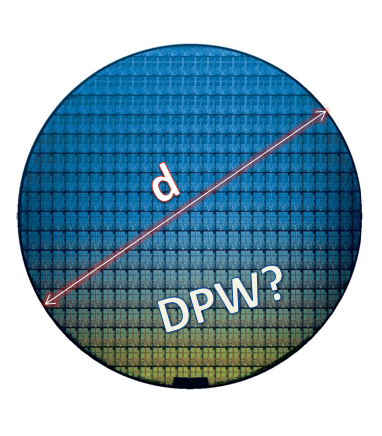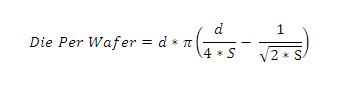Die Per Wafer Formula and (free) Calculator

April 09, 2013, anysiliconCalculating the number of Dies Per Wafer (DPW) is a very simple and straight forward task. It’s actually based on basic high school mathematics which are related to circle area formula, remember Pi?

Silicon dies which are placed on a wafer can also be described as many squares placed inside a circle — thus the calculation is about first finding the overall circle area using both the mathematical number Pi (approximately equal to 3.14159) and the wafer size.

The wafer size and the die size are known in advance, however, as our “squares” have spaces between them (e.g. scribe lines) and the area located at the edge of the wafer cannot be used, the calculation is a bit tricky, therefore, some recommend using the Die Per Wafer tools results as an estimation rather than a calculation.In addition to the above unused area, the foundry will use additional area for testing purposes (PCM structures) that will eat up relatively small size of the wafer. Sawing lanes, wafer margin and test structures size vary from process node to process node and from foundry to foundry. Therefore, is it highly recommended to have the final DPW figure directly from the foundry because they have all the knowledge and information required to provide the actual figure.

AnySilicon’s Die Per Wafer free Tool

Our free Die Per Wafer calculator is very simple and based on the following equation:d – wafer diameter [mm] (click her for wafer size information)

S – die size [square mm]

For your convenient, we have placed the Die Per Wafer calculator as an online Excel sheet so you can use it online or download it into your ASIC price calculator.

mm
mm
µm
µm
mm
mm2
mm2

To make life a bit easier we are offering a simple downloadable Excel calculator that helps calculate die per wafer for different wafer and die size. Download free DPW Calculator

What’s next?

Get price quote for ASIC design services here.

Or get wafer price proposals from vendors here.